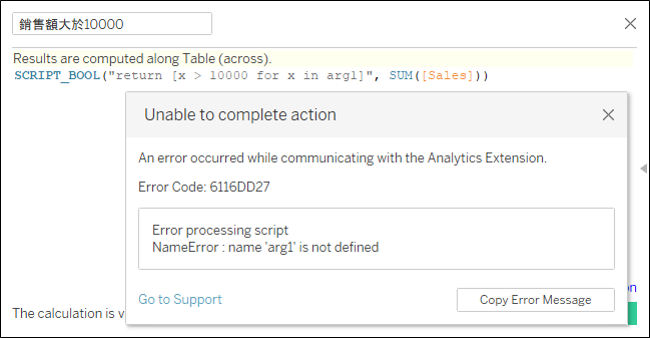#DAY 27
0
AI & Data

## 詳細錯誤訊息``````[INFO] : function to evaluate=def _user_script(tabpy, _arg1):
return [x > 10000 for x in arg1]

[ERROR] : Responding with status=500, message="Error processing script", info="NameError : name 'arg1' is not defined"
[ERROR] : 500 POST /evaluate (::1) 3.99ms
``````

``````def _user_script(tabpy, _arg1):
return [x > 10000 for x in arg1]
``````
• `_user_script(tabpy, _arg1)`：第一個參數 `tabpy` 不是我們所關心的，因此可以從第二個參數開始看。這裡的參數 `_arg1` 是不是很眼熟？就是在 [Day24] Tableau 輕鬆學 - TabPy 使用方法 1 提到的，TabPy 預設會以 `_arg#` 變數名稱來存取資料，就是因為這裡的 `_user_script` 函式所定義，而我們在 Tableau Desktop 所寫的 Python 程式會變成 `_user_script` 的本體。

## 傳輸資料查看

`銷售額大於10000` 運算函式修改為

``````SCRIPT_BOOL("print('Hello TabPy')
return [x > 10000 for x in _arg1]", SUM([Sales]))
``````

``````[INFO] : function to evaluate=def _user_script(tabpy, _arg1):
print('Hello TabPy')
return [x > 10000 for x in _arg1]

Hello TabPy
``````

``````SCRIPT_BOOL("print(_arg1[:10])
return [x > 10000 for x in _arg1]", SUM([Sales]))
``````

``````...
...
...
[INFO] : function to evaluate=def _user_script(tabpy, _arg1):
print(_arg1[:10])
return [x > 10000 for x in _arg1]

[1270.53]
[INFO] : function to evaluate=def _user_script(tabpy, _arg1):
print(_arg1[:10])
return [x > 10000 for x in _arg1]

[1603.1360000000002]
``````

Tableau 輕鬆學30